# Frame 3  - Complex Geometry

## The Hex-Machine              Three generations of hexagons based on the Athena engraving's boundary points

The Frame teaches geometry: two dynamic star systems, so far, in which stars rise from other stars. One is an intricate design made of three generations of hexagonal stars.  We remember the Strong Connection (B-G) and the Tri-balance (EFGH) which stole the show in the previous chapter. Here, the same agents dominate in the hexagonal arragement below, the Big Hex.  The star shares centers with the Main Square; the fact is pretty well a guarantee of an exact relationship between the Cone & Square configuration and the Hex-Machine. However, I haven't yet discovered it.
Be it as it may, it's undeniable that the design has theoretical, didactical value, for it concerns itself with some finer points of a classical position from geometry.a) Rounded to the nearest degree, The angle F-G-H measures 120 degrees.
b) The line G-B then divides
F-G-H into two 60 degree angles.
c)
G-B passes through the center of the Main Square with faultless accuracy. In the diagram, the Square's axes are represented by the red cross hairs within the small circle.

d) The lines connecting the Square's center (the 0,0 point) to 'E' and 'G' hold together a 60 degree angle. They create a close facsimile of an equilateral triangle with the extension of F-G, as below.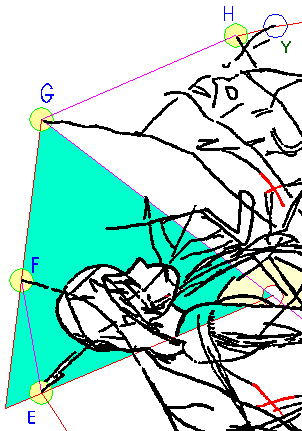The Big Hex

So, we have some lines
and an equilateral triangle which could all be part of one regular hexagon. So, why not draw it? The result is seen in the first diagram; there is splendid fit between the big hexagon and the engravingFor instance, the hexagon's circumcircle covers the image remarkably well. The same can be said for the envelope around the human figure.

I noticed the Big Hexagon first, because it is so obvious. Its origin remains unknown until one understands two other 60 degree angles in this position, how they work together. Several experiments have to be carried out.

The basic array of the 60 degree angles on the Frame is as below.Each line from B to one of the four points  E,F,G,H serves as an arm of at least one 60-degree angle (rounded out to the nearest degree).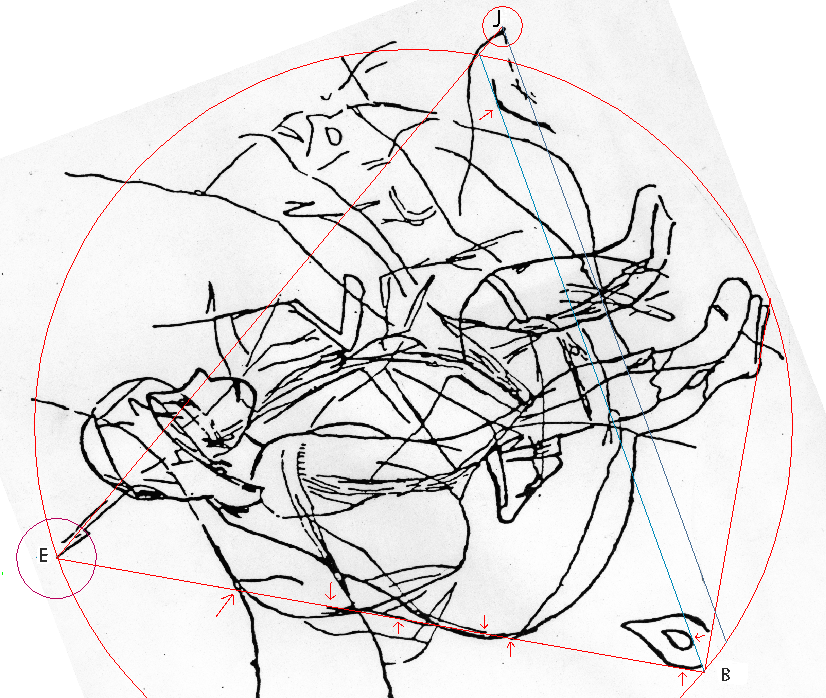If we rotate E-J clock-wise 60-degrees around 'E' - it turns out that the new line can always be easily recreated  without measurement, just relying on line-guides (marked by red arrows) supplied by the engraving. Note that the line JB doesn't actually hit 'B'. Instead, it subtends a section of the engraving very cleanly.  Next, we complete the angle JEB into an equilateral triangle by resting the third side on the Frame-point 'B' (diag. above).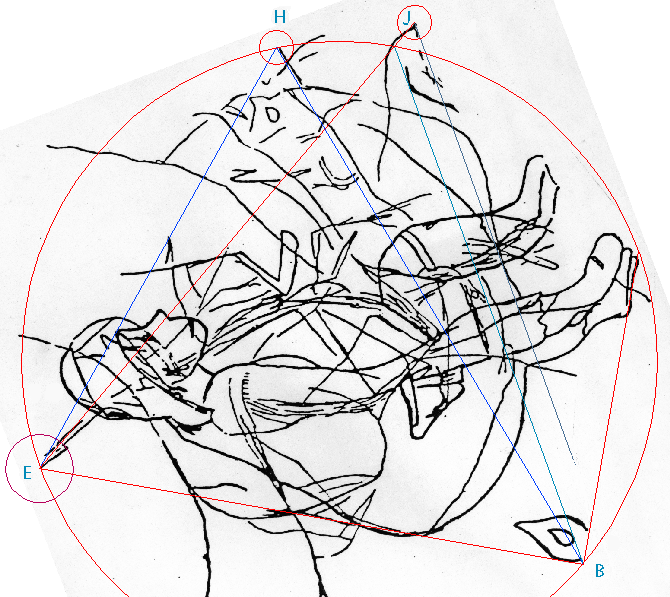The triangle's circumcircle then touches 'H' (above). Moreover, lines from 'E' to 'H', and 'H' to 'B' also create an angle which rounds out to 60 degrees.  The position is now an illustration of a geometrical theorem: Any point on the circumcircle of an equilateral triangle connects to that triangle's corners by lines holding 60 degrees when above the basis, and 120 degrees, when below the basis. The point H happens to be just such a point. So, it must have been drawn with the red triangle already in place. (diag. below) It also seems that B-H was drawn from 'B', so that it would pass through 'P', a point on the Red Hexagon.  Next, complete E-H-B into an equilateral triangle set by E-H, which otherwise subtends the Tri-balance.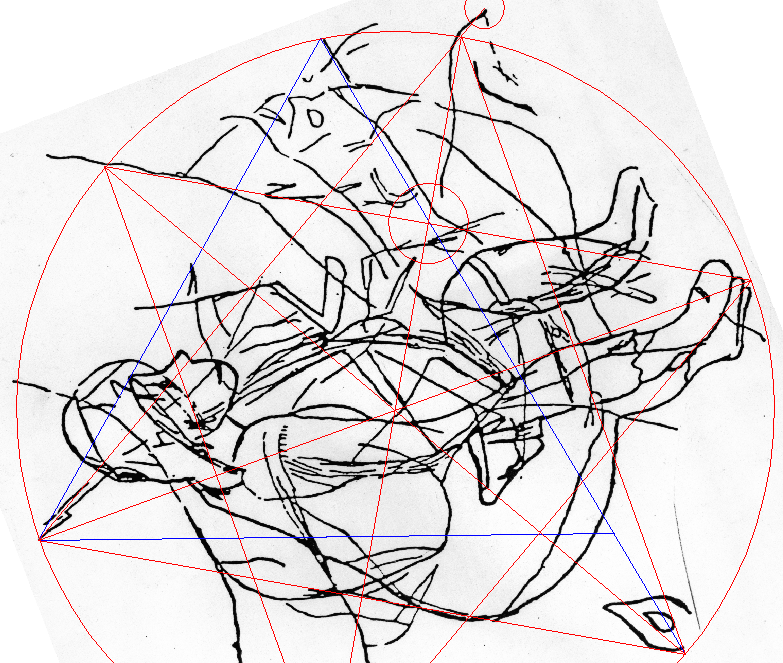(Above)  Both the red and blue figures are perfectly regular, but their coordination is slightly imperfect in illustrating a special case. (Below)  Perfecting the positional idea brings the blue figure visibly away from the H point. Each of the thirteen Frame points serves many masters (functions), thus, not all can be served ideally. What matters is that exact ideas can be effectively communicated by the language of approximations, and that's the case here.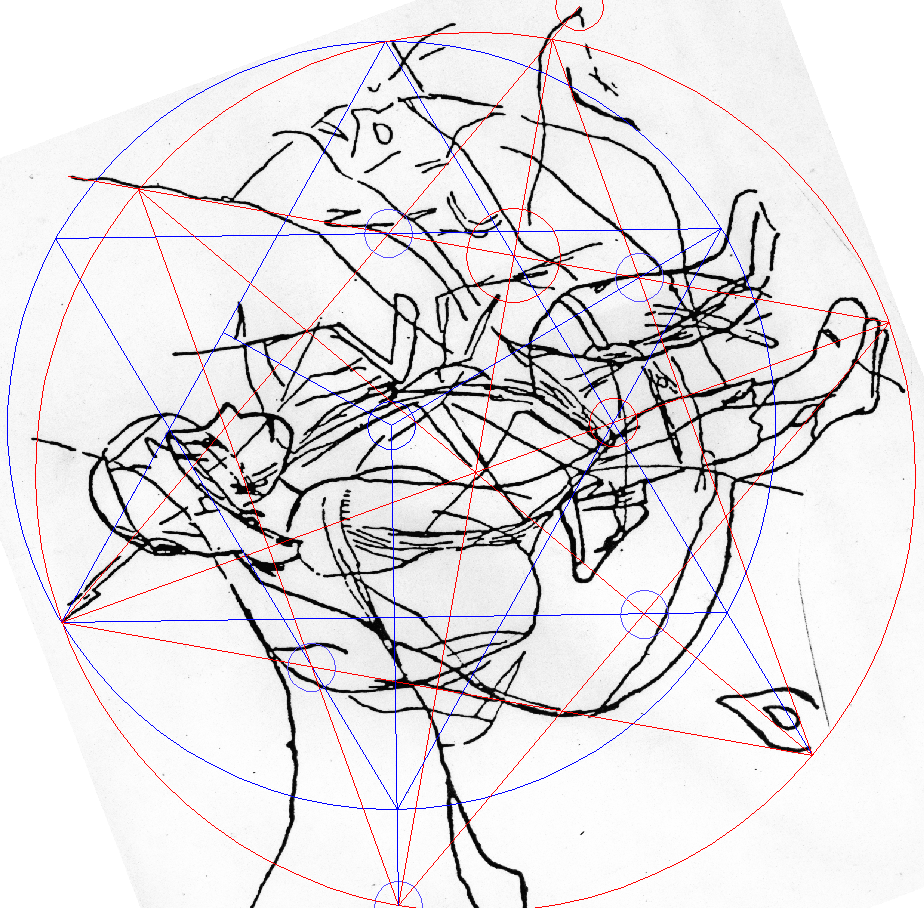A Special Case

Here is the design with more perfect points in common (circled) to the two stars
without interference of the engraving's background.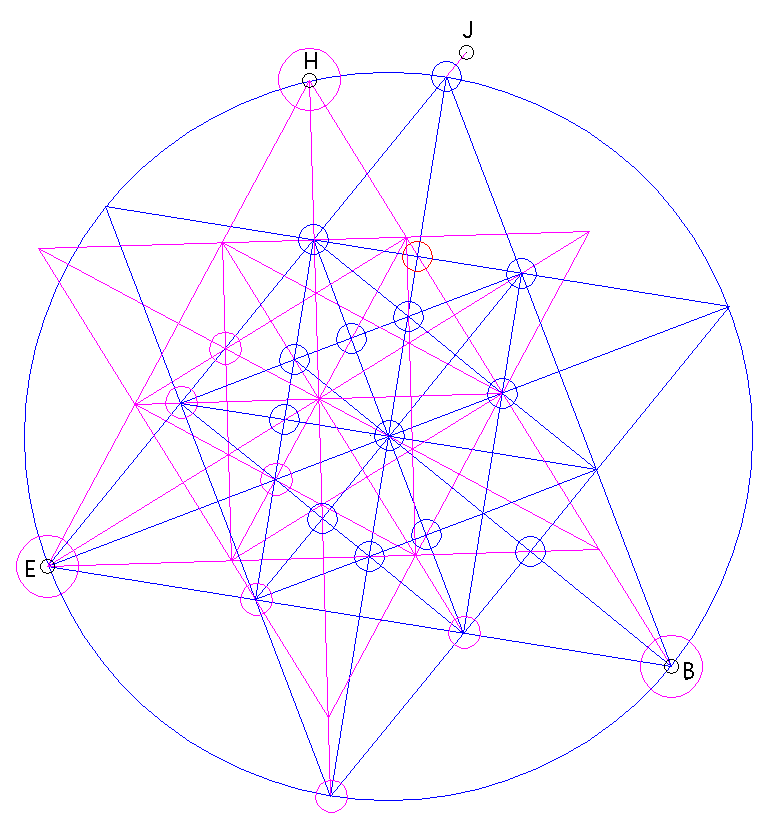Any changes in the position of "F" on the circumcircle eliminate most, but not all, of the perfect common points.  Clearly, the position is more than just an example of the basic theorem; it deals with

a special casefurther refining that theorem. This special case occurs only when
the line B-H ( or its mirror image ) passes through the circled point
on the other figure, as in the diagram above (at 1/4 of the circle's diagonal,
1/3 of the triangle's height).

# The above image shows the three figures as given by the engraving: the Red Hex, the Blue Hex, and the Big Hex. Each figure is geometrically perfect per se but slightly off perfection in relation to the other two at portraying exact relations found among them. This imperfection is clearly visible at the crucial point 'B', where the magenta-coloreddiagonal of the Big Hex should pass exactly through the point, which the other two stars have in common.  That said, one has to marvel at how the image above gives us a perfect picture of what is going on; it is an accurate depiction of exact ideas. Points that should be perfect exist instead in tightly confined spaces sometimes bordering on invisibility.The Big Hexagon is third-generation! The first hexagon found on the Frame - the Big Hex and the other two hexagons coincide at various points. Could it be that like the second hexagon is a special case of the first hexagon, the Big Hexagon is a special case based on both? In the diagram above, we see how the Big Hexagon fits together with the orange star - the Hex Machine's first generation. The diagram below shows the Big Hexagon and the Hex Machine's second generation, and their points of exact coincidence.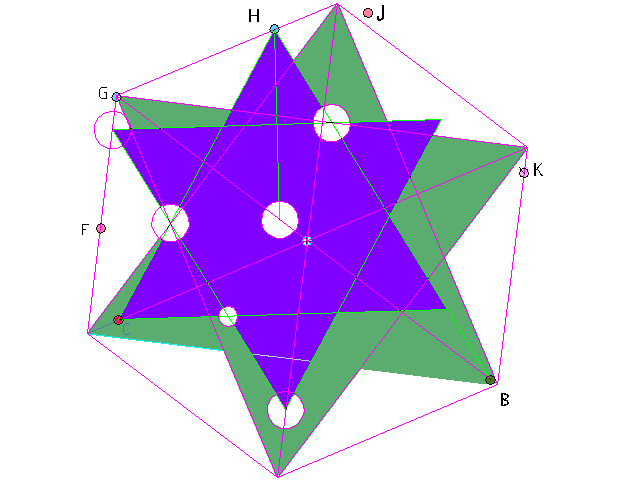Take those ideas and put them together, and you get the big picture.

All three hexagonal system together become the Hex Machine.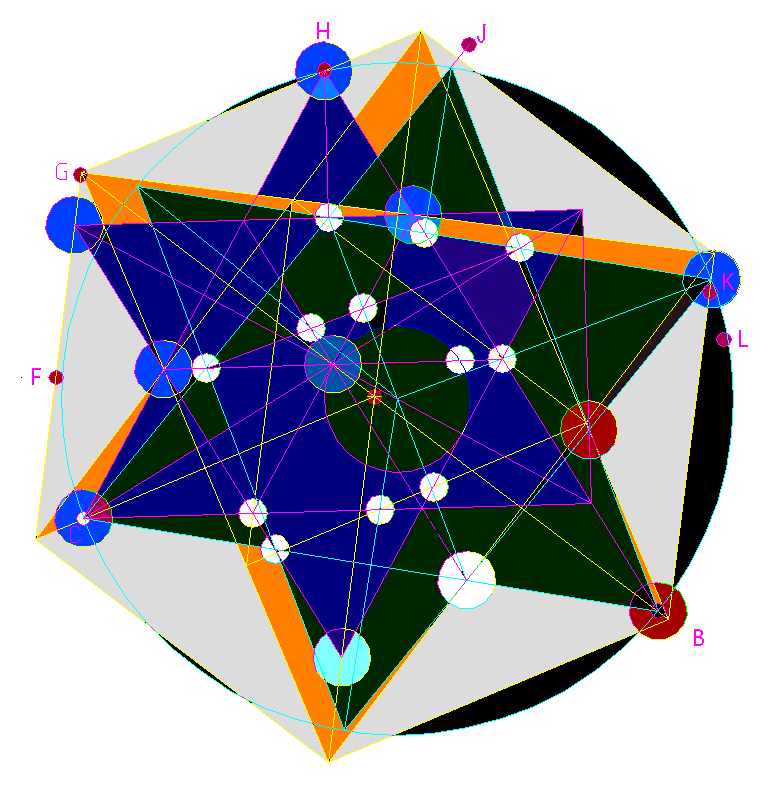It's time again to review the standard argument against research like this - that one can find some wonderful geometric order in anything -  bicycles, dimensions of cereal boxes, pieces of wind-blown  newspaper, etc. Here, that argument holds no water; the Frame exemplifies a different kind of order. It's highly intelligent, no doubt about it.

(C) Jiri Mruzek